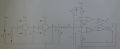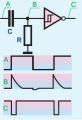# What is function of this complicated circuit?

#### xxxyyyba

Joined Aug 7, 2012
289

a) Explain principle of working of circuit below. Draw waveforms of voltage at points A, B, C, D and Q, as well as capacitors voltage waveforms.
b) What is minimum time constant of charging of C3 (ie value of resistance R6) so that voltage waveform of point Q are rectangular pulses

It is known: R1, R2, R3 (R2=R3), R4, R5, R6, C1=C2=C3, 5R1>>R2 and R1>>R3. Threshold voltage equals VDD/2.Any suggestion?
I did nothing because I have no idea how to startI'm beginner in Digital electronics. In analog electronics and in circuit theory, there are well known methods for solving circuits, but what to do here? I'm really stuckEDIT: This is not homework question. I don't have final result. This was on my exam. I didn't solve it on exam and I'm trying to figure out what is solution to this problem. Every suggestion is welcome, I want to pass exam next timeLast edited:

#### Jony130

Joined Feb 17, 2009
5,435
First notice that the NAND gates work here as a inverter (NOT gate) and this two NAND gates form almost a classic example of a astable generator.
And the right part of a circuit (Two op amp + NOR gate SR flip-flop and BJT) is a slightly modified version of the famous NE555 IC.

Also this diode across R6 is wrongly connected

•xxxyyyba

#### xxxyyyba

Joined Aug 7, 2012
289
Hello, thanks a lot for reply. So original "complicated" circuit consists of few simple circuits conected together and to understand "complicated" circuit, I should first understand its "parts", right?
But then it's not clear to me: Let's say I analysed first part of this circuit (astable generator) and found voltage waveform at point B. If I then connect second part to it (high pass filter - C2R4), it would "interact" with previous stage and, logically, waveform of point B I got when analyzed first stage on it's own will CHANGE?

Last edited:

#### Jony130

Joined Feb 17, 2009
5,435
Point B voltage is a GATE output which means that this voltage is load independent (C2 and R4 will not have any influence on the voltage at point B ).
In fact the voltage at point B will be a square wave with amplitude equal to VDD because gate output will behaves just like a voltage source.•